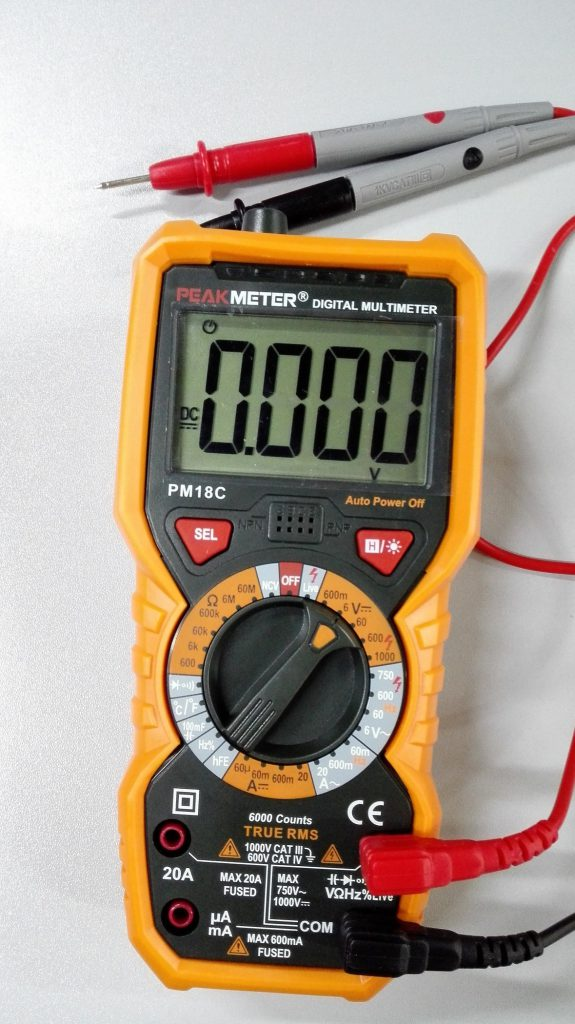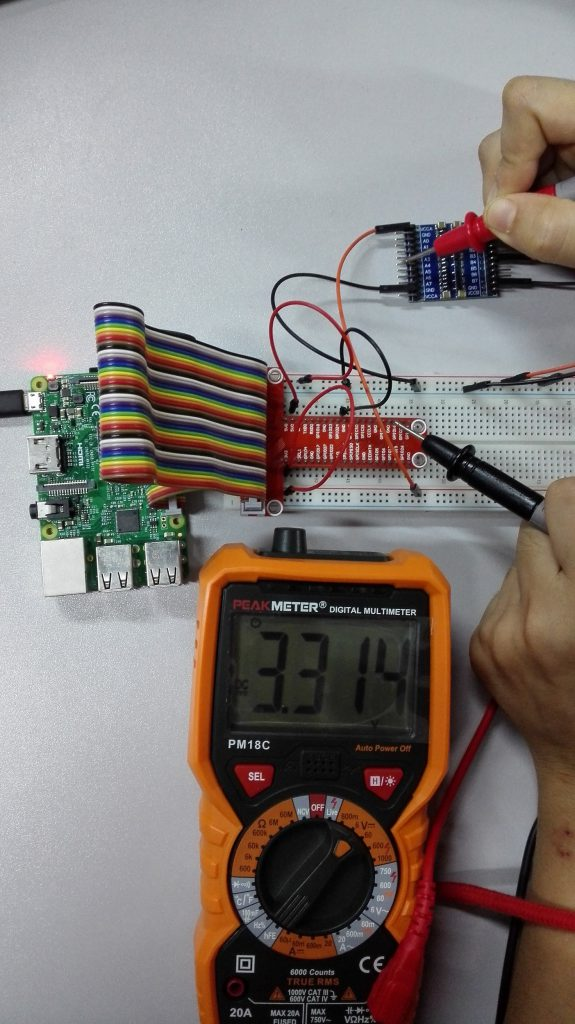### Overview

Raspberry Pi GPIO pin  working voltage is 3.3v.  However, many sensors and devices (especially those designed for Arduino) work at 5V.  The 8 Channel Logic Level Converter is a bridge to connect 3.3V i/o pins to 5V pins. 3.3v signal input to Ax pins can automatically be converted to 5v output to Bx pins and 5V input signal to Bx pin can also be covert to 3.3v to its counterpart Ax pins.

In this project, we will show how to this 8 Channel Logic Level Converter convert Raspberry Pi 3.3V signal to 5V output.

### Features

• 8 channels bidirectional voltage translation between different logic level, translate between Ax and Bx automatically
• 4 pairs of power supply interfaces, supports more situations
• Voltage level: 1.8V-6V

Hardware Preparation:

1 * Raspberry Pi
Some Jumper wires
1 * T-Extension Board
1 * 8 Channel Logic Level Converter
1 * multimeter

### Test

Assembling the circuit as followed Connection Graph:• VCCA/VA connects to 3.3V power supply
• VCCB/VB connects to 5V power supply
• GND connects to power negative pole respectively, the two power supply should be common-grounded with each other
• When Ax has TTL 3.3V input, Bx will get TTL 5V output
• When Bx has TTL 5V input, Ax will get TTL 3.3V output

In this example, we will connect B4 to 5V, then use multimeter to test A4 output voltage.

First, set the multimeter to “6V” in the DC range (Different Multimeters has different scale. You have to set the multimeter to a range that it can measure.  here we need to measure 3.3v, so we set to 6V which can measures voltages up to 6 volts)Connect the black probe to Raspberry pi “GND” and the red probe to 8 Channel Logic Level Converter “A4”If you set it incorrectly, you will probably see the meter screen change and then read about ‘3.3’.  This means the 8 Channel Logic Level Converter has converted signals from 5V down to 3.3V.